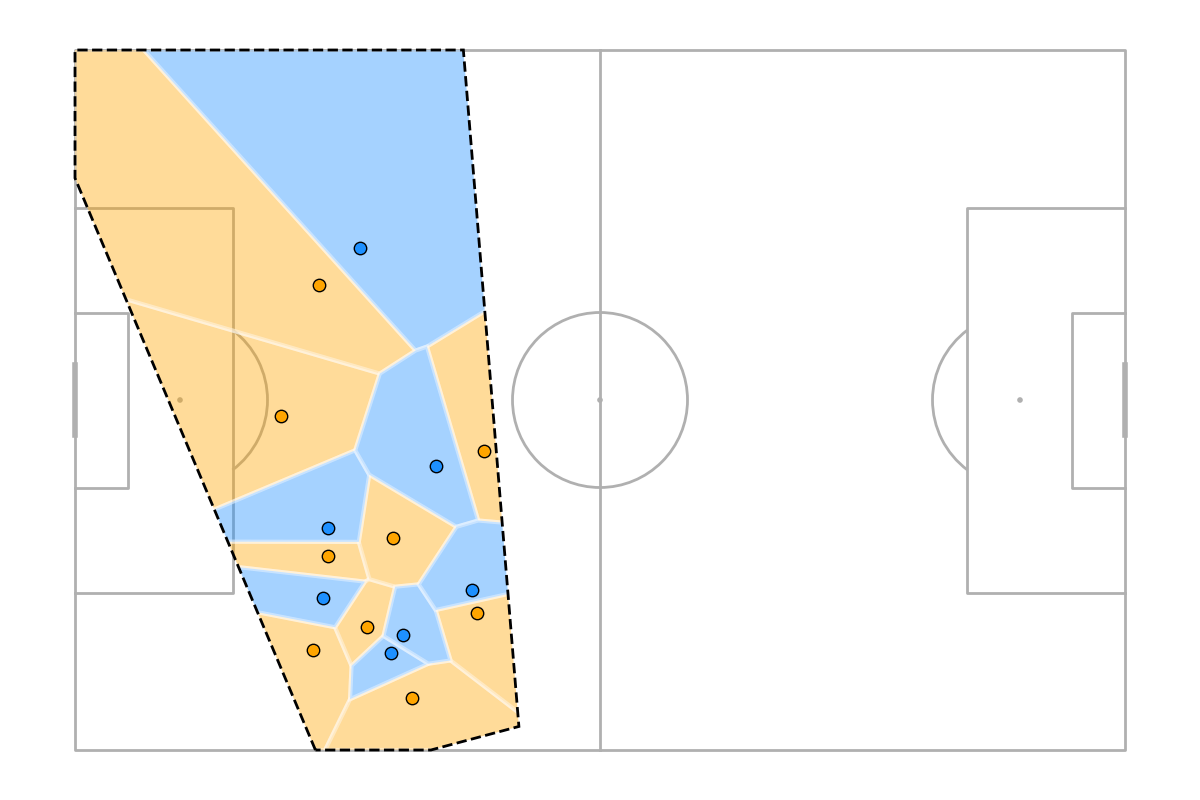# Plots a Voronoi diagram¶

This example shows how to plot a Voronoi diagram for a shot freeze frame.

```import matplotlib.pyplot as plt
import numpy as np
import pandas as pd

from mplsoccer import VerticalPitch

plt.style.use('dark_background')

# get event and freeze frame data for game 7478
tactics_lineup_df=False, warn=False)
df_event = dict_event['event']
df_freeze = dict_event['shot_freeze_frame']
```

Subset a shot

```SHOT_ID = '8bb8bbc2-68a6-4c01-93de-53a194e7a1cf'
df_freeze_frame = df_freeze[df_freeze.id == SHOT_ID].copy()
df_shot_event = df_event[df_event.id == SHOT_ID].dropna(axis=1, how='all').copy()
```

Location dataset

```df = pd.concat([df_shot_event[['x', 'y']], df_freeze_frame[['x', 'y']]])

x = df.x.values
y = df.y.values
teams = np.concatenate([[True], df_freeze_frame.player_teammate.values])
```

Plotting

```# draw plot
pitch = VerticalPitch(half=True)
fig, ax = pitch.draw(figsize=(8, 6.2))

# Plot Voronoi
team1, team2 = pitch.voronoi(x, y, teams)
t1 = pitch.polygon(team1, ax=ax, fc='#c34c45', ec='white', lw=3, alpha=0.4)
t2 = pitch.polygon(team2, ax=ax, fc='#6f63c5', ec='white', lw=3, alpha=0.4)

# Plot players
sc1 = pitch.scatter(x[teams], y[teams], ax=ax, c='#c34c45', s=150)
sc2 = pitch.scatter(x[~teams], y[~teams], ax=ax, c='#6f63c5', s=150)

plt.show()  # If you are using a Jupyter notebook you do not need this line
```Total running time of the script: ( 0 minutes 1.208 seconds)

Gallery generated by Sphinx-Gallery